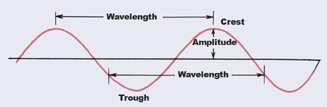A# waveFigure 1. In longitudinal waves (A) the medium in which the wave is traveling moves backward and forward in the direction in which the wave is traveling (for example, the movement of air as sounds travel through it). In transverse waves (B) the motion of the medium is at right angles to the direction in which the wave travels (such as the movement of water waves). Electromagnetic waves (C) are transverse waves set up by high frequency electric and magnetic forces interlinked and acting at right angles. They can travel without a medium (such as light). To examine a wave the wave must be converted to some other form of energy: Sound waves cause (D) a microphone diagram (1) to vibrate, compressing the carbon granules (2), so altering the resistance between the blocks (3). A water wave can cause light to be focused onto a screen (E); a light wave can cause a material in a photocell to give up electrons and hence set up a current.Figure 2. Components of a wave.

A wave is a disturbance that travels from one place to another without transporting any matter. It may or may not transport energy (see below). The source of all waves is something that is vibrating, moving back and forth at a regular, and usually fast rate.

## Types of wave

Waves may be longitudinal or transverse (see Figure 1). In longitudinal waves the medium moves back and forth along the direction in which the waves are traveling. Sound is an example of a longitudinal wave, being carried through air the by a wave motion in which the air molecules vibrate parallel to the direction of propagation. A wave traveling down a Slinky is also longitudinal..

In transverse waves the motion of the medium is at right angles to the direction in which the wave travels. Familiar examples of transverse waves are the surface waves on water, or the wave that can be made to travel down a stretched rope. Light and other forms of electromagnetic radiation involve electromagnetic fields oscillating perpendicular to the direction in which the wave propagates.

### Standing wave

A standing wave, or stationary wave, is one in which the nodes and antinodes – points of zero and maximum amplitude – appear not to move. A standing wave is formed by the overlapping, or interference, of traveling waves of equal frequency and intensity moving in opposite directions.

## Characteristics of waves

Waves have high points called crests and low points called troughs. The vibration between two extreme points – the crest and the trough – is generally an "oscillation."

Amplitude refers to the maximum distance on either side of the midpoint of the wave (see Figure 2). The distance from the top of one crest or tough to the next is called the wavelength. How frequently a point on a wave passes a particular point per unit time is described by its frequency. The unit of frequency is the hertz (Hz) after Heinrich Hertz. Once per second is 1 hertz, twice per second is 2 hertz, etc. The product of the wavelength and the frequency gives the velocity of propagation of the wave.

The time it takes for an object to make a complete vibration – for instance, the time it takes a pendulum to swing once each way – is called its period. Frequency and period are reciprocals of each other.

According to the direction and form of the local oscillations of the medium, different polarizations of the wave are distinguished.

The characteristic properties of waves include propagation in straight lines; reflection at plane surfaces; refraction – a change in direction of a wave transmitted across a plane interface between two media; diffraction – diffuse scattering by impenetrable objects of a size comparable with the wavelength; and interference – the cancellation of one wave by another wave half a wavelength out of step (or phase) so that the crests of one wave fall on the troughs of the other.

If the wave velocity is the same for all wavelengths, then quite arbitrary forms of disturbance will travel as waves, and not simply regular successions of crests and troughs. When this is not the case, the wave is said to be dispersive and localized disturbances move at a speed (the group velocity) quite different from that of the individual crests, which can be seen moving faster or slower within the disturbance "envelope," which becomes progressively broader as it moves.

Waves carry energy and momentum with them just like solid objects; the identity of the apparently irreconcilable wave and particles aspects of matter is the basic tenet of quantum mechanics.

## Wave front

A wave front is a contour on which at every point the medium or electromagnetic wave is vibrating in step (that is, in phase). The wave front is usually at right angles to the direction of wave motion, and may be plane, spherical, or otherwise. Each point is a source of secondary wavelets, which give rise to a new wave front position a short time later.